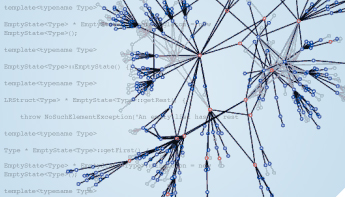# Neumont Coding Contest 2015 - Qualifying Round Solution 4:06 PM Algorithms , Coding Contest 0 CommentsCourtesy University of Buffalo

## Beware! Solutions follow! First, read the Betsy Ross problem and see if you can come up with your own solution.

Here is one of the submitted solutions to the Betsy Ross problem posted in the previous blog post:

``````
public class BetsyRoss {

public static void main(String[] args) {
Scanner input = new Scanner(System.in);

String line = input.nextLine();
Integer starCount = Integer.parseInt(line);

double bestColumnCount = starCount;
double bestRatio = starCount;
for ( int n = starCount - 1; n >= 1; n-- ) {
int remainder = starCount % ( n + ( n - 1 ) );
if ( remainder == 0 || remainder == n ) {
double rowCount = starCount / ( n + ( n - 1 ) ) * 2 + (remainder == 0 ? 0 : 1);
double ratio = Math.max(n, rowCount) / Math.min(n, rowCount);
if ( ratio < bestRatio ) {
bestColumnCount = n;
bestRatio = ratio;
}
}
}

System.out.println((int)bestColumnCount);
}
}
``````

### Math

Amongst the fastest-submitted solutions, the most important realization for students was that the odd/even complexity could be reduced by envisioning the rows in odd-even pairs. Either a flag would be comprised of all odd-even pairs (or even-odd pairs) or there would be one odd (or even) row left over.

This means that if one is testing with seven starts on the top row, the flag can be thought of in 13-star groups (7 + 6) with a possible 7 left over. This means that if the total number is evenly divisible by 13 or if it has a remainder of 7 (meaning one additional odd row), then we have a match.

Note that the same principle works if the first row has an even number of stars on the top row. In the case of 8 stars on top, the flag can be though of in 15-star groups (8 + 7) with a possible 8 left over. This means that if the total number is evenly divisible by 15 or if it has a remainder of 8 (meaning one additional row), then we have a match.

Reducing this step of the problem to mere mathematics saves us inner loops that might need to discover the fact empirically, making the solution faster and writable in fewer lines of code.

### Max

Another simple construct in problems like this is a max counter. The problem set stated that the number of rows and columns needed to be as close to identical as possible. When looping through experiments, one can keep track of the current best, updating it only when a better one comes along.

### Improvements?

Please post below your improvements! The above code runs in under 5 seconds on nine-digit numbers on my machine.

"I love to teach, as a painter loves to paint, as a singer loves to sing, as a musician loves to play" - William Lyon Phelps